Smartick is a fun way to learn math!Sep30

# The Associative Property and Mental Math

In today’s post, we will see how useful the associative property can be when we want to do mental math with addition and multiplication.

To begin, I would recommend that you review these earlier posts from our blog about the associative property that could be very useful:

Have you taken a look? Great, now that you have refreshed your memory we can get down to business.

We begin by looking at an example with addition: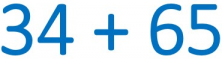It can be difficult to complete the equation outright, but we can make it easier by using the associative property as shown below:

• First, we decompose or break down, the numbers. Try to break down one of the addends into numbers that will be easy to solve mentally.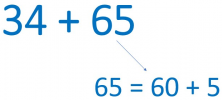• Then break everything down as shown in the following image: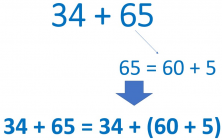• Now you only need to apply the associative property and solve: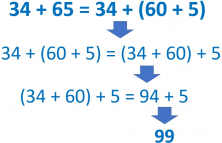• And there you have it!

34 + 65 = 34 + (60 + 5) = (34 + 60) + 5 = 94 + 5 = 99

You can see that 34 + 65 is 99.

## Associative Property of Multiplication

Now we will look at another example, this time using multiplication: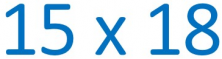It seems difficult but in reality, we just do the same thing as we did with addition.

• The first thing to do is to break down the factors so that we are left with a simple equation that we can solve mentally.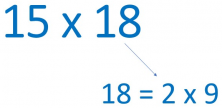• Then, put everything together as seen in the following image: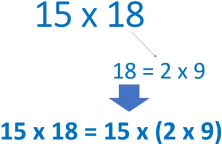• And finally, apply the associative property and solve: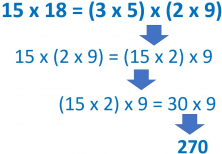• And that’s it!

15 x 18 = 15 x (2 x 9) = (15 x 2) x 9 = 30 x 9 = 270

You can see that 15 x 18 is 270.

Now you will find it much easier to solve problems with mental math. Practice what you’ve learned and you’ll see!

I’ll leave you with some equations so you can continue practicing, but you can also make some up yourself and then check that you have solved them correctly.

• 67 + 42
• 31 + 58
• 35 x 16
• 25 x 24